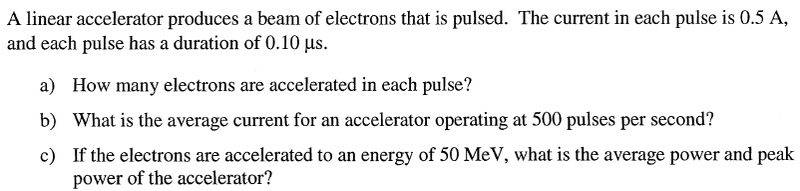# Pulsing beam of electrons

## Homework Statement## The Attempt at a Solution

I'm at a loss as to starting the problem. Any help is appreciated!

gneill
Mentor
What is the relationship between current and charge?

i = dQ/dt, but I can't figure out how to derive/integrate here :(

gneill
Mentor
i = dQ/dt, but I can't figure out how to derive/integrate here :(

No integration is required. You're given the current (0.5 A) and the time that it flows for each pulse (0.10 μs). If 0.5 A flows for 0.10 μs, how much charge has moved?

Thank you very much, I forgot the elementary relation Q = it. I got 3.1 * 10^11 electrons for a) which is the right answer :)

For part b), do we just divide average current per pulse by number of pulses per second?

gneill
Mentor
For part b), do we just divide average current per pulse by number of pulses per second?

The average current will be given by the total charges moved in a second, divided by one second. You've found the charge q moved per pulse, and you know the number of pulses per second, so...

Yup, got it. Part c) ?

gneill
Mentor
What are the units of power? What's the energy per charge? Per pulse?

Again, I have forgotten that electrons having an energy of 50 MeV have been accelerated across 50 MV. Thanks!